# Structured Types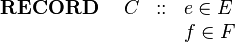$\begin{array}{lcl} \textbf{RECORD}~~~~ C &::& e\in E\\ && f \in F \end{array}$
We can model this structure in Event-B by introducing (in a context) a set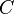$C$ and two functions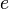$e$ and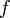$f$ as constants as follows: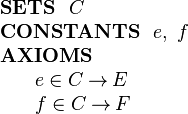$\begin{array}{l} \textbf{SETS}~~ C\\ \textbf{CONSTANTS}~~ e,~ f\\ \textbf{AXIOMS}\\ ~~~~\begin{array}{l} e \in C \tfun E\\ f \in C \tfun F\\ \end{array} \end{array}$
Now, given an element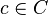$c\in C$ representing a record, we write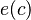$e(c)$ for the 'e' component of structure